## Mentors

• Pranav DV
• Sanjkeet Jena

• Samhita R
• Vikas Bhatt

## Introduction

The objective of this project was to recreate the popular solved game “Connect-4”. We created both singleplayer and multiplayer modes with the single player mode being played against AI. The AI was constructed using minimax algorithm and optimized using alpha beta pruning. The graphic user interface was made using a python graphics library called Pygame.

## Implementation

The first stage of our project was to make a simple CLI version of 2 player connect 4.

The first step to do so is make a game board. We have used an integer array with 6 rows and 7 columns as the game board. The number 0 represents an empty cell, 1 represents tokens of the first player and 2 represents tokens of the second player.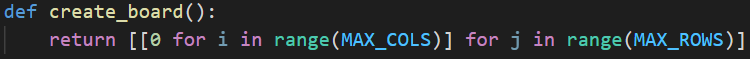We also define a print_board() function here to display the board to the user in the command line terminal. The function iterates through the board and prints the value in each cell.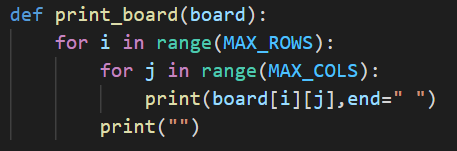The next task is to take input from the user and insert their piece in the game board. We do so in the following way:

1. Check if the column entered by the user has any empty cells. We define the valid_choice() function which checks if the topmost cell of the entered column number is empty or not. It does not need to check other cells since pieces fall to the bottom in the vertical Connect 4 board. If the entered column is full, a message is printed on the screen.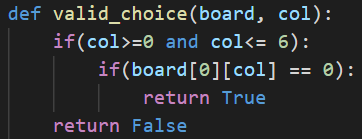2. If the entered column is valid, then we get the lowest empty cell in that column using the get_row() function. It iterates through the cells to find the required cell.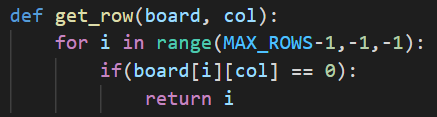3. Change the value of the cell to the user's value. The row and column numbers of the cell are known to us. To find the users value, we define a variable called MOVES, which tracks the number of moves made in the game. When it is odd, it is the second player's move, and when it is even, it is the first player's move. It also helps us to determine if the game ends in a draw as we will see later. MOVES is incremented after each move.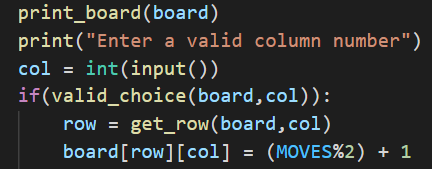Finally, we must determine when the game ends and print an appropriate message. The game ends when one of the players win, or if the board becomes full, in which case it is a draw.

To determine if a player has won the game, we define a function winning_check() to iterate through the board and check each row, column and diagonal for 4 in a row. It returns 0 if neither player has won, otherwise it returns the winning player's number.

Following is a section from the winning_check() function: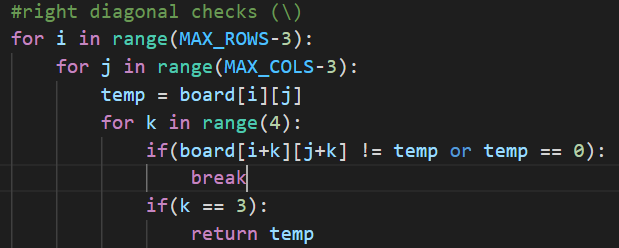To know if the game ended in a draw, we check the MOVES variable. There are 7 x 6 = 42 cells in the board. When the MOVES variable becomes 42, the board is full, and the game ends in a draw provided that neither player has won.

We finally get the following code: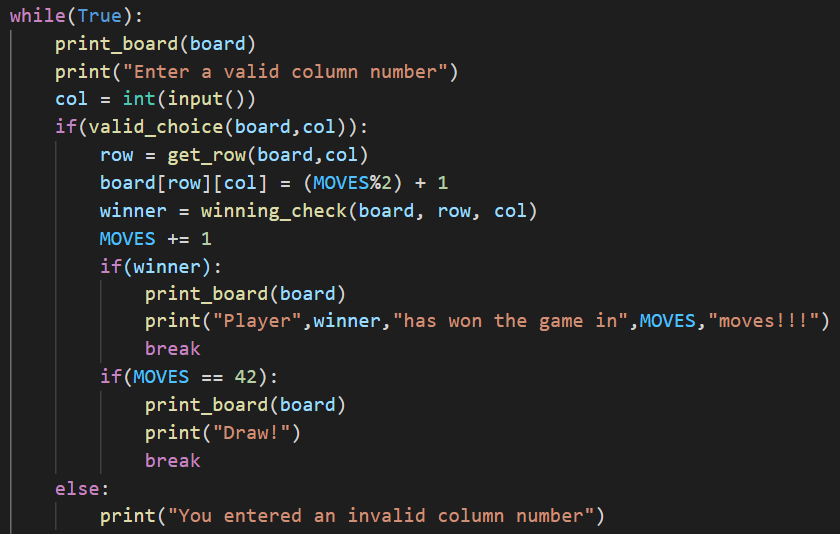The final 2 player CLI game is shown below: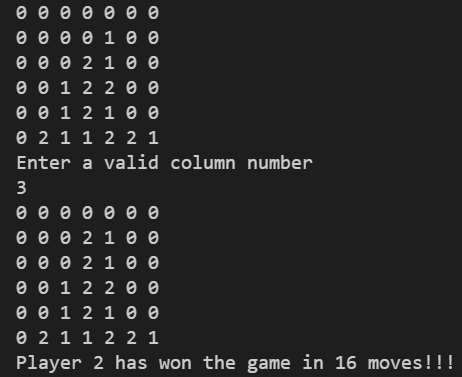## Minimax Algorithm

This was used to implement the single player version of the game, i.e, the user playing against the computer. The minimax algorithm explores all possible moves in a game tree upto a specific depth and returns the most favourable move to the computer.

Concept: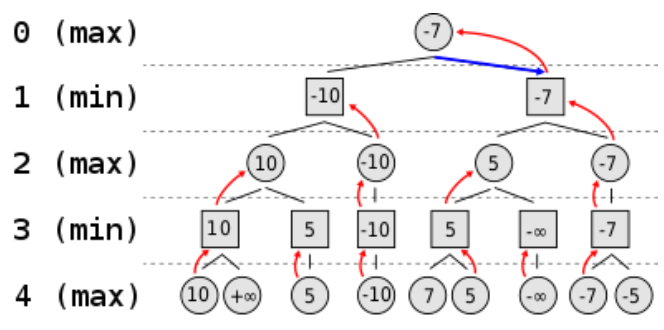The core concept of this algorithm can be explained using this game tree.

At each level the maximum/minimum value of the child nodes is chosen depending on whether the player is maximizing or minimizing. For example, at level 3, the first node is assigned 10 over infinity as it is the minimizing player’s move. At level 2 the first node is assigned 10 over 5 as it is the maximizing player’s move. In this fashion the algorithm assigns values to all nodes of the game tree upto a specific depth using depth first search and recursion. The value assigned to the root node represents the most favourable move the computer can make.

Implementation: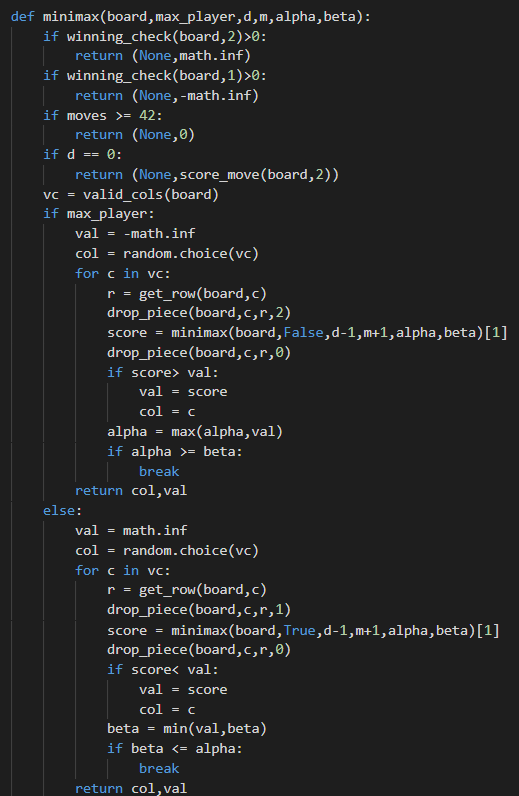The minimax algorithm in our game is implemented using the recursive minimax function which takes the depth, maximizing player and number of moves as parameters and it returns the most favourable column according to the highest score. In case the maximizing player has won, the function returns the maximum possible value infinity and returns negative infinity if the minimizing player has won. In case the game is a draw, zero is returned. In case the depth passed to the function is zero then the overall score calculated for the current board by the score_move function is returned. In all of these cases no column is returned since no further moves can be checked by the function. All these cases form the base cases of the function.

In case none of these conditions are satisfied, the function checks whether the player passed to it is the maximizing player or not and gets a list of all the valid columns where moves can be made using the valid_cols function. If the player is maximizing, the initial value is set to negative infinity. Then a loop is run considering all possible moves by dropping the token in each column one by one. The minimax function is then called on it’s child node and the score obtained is compared with the current value. The maximum of these two is set to be the value for the next iteration. The maximum value obtained after iterating through all columns, i.e, after going through all moves and the column which gave that value, i.e, the move which yielded that value is then returned. In case the player is minimizing the initial value is set to positive infinity and a similar loop is run considering all possible moves and the least value obtained after iterating through all columns and the respective column which yielded that value are returned.

The overall score is evaluated using the evaluate and score_move functions. The score_move function checks each row, column, positive and negative diagonals for the tokens present in them in windows of 4 slots each. Each window is passed to the evaluate function which returns the score of a particular window. The center column is also given priority in this function since it is easier to construct rows, columns and diagonals of four using tokens from the central column.The overall score of a particular move after considering all possible windows is then calculated and returned.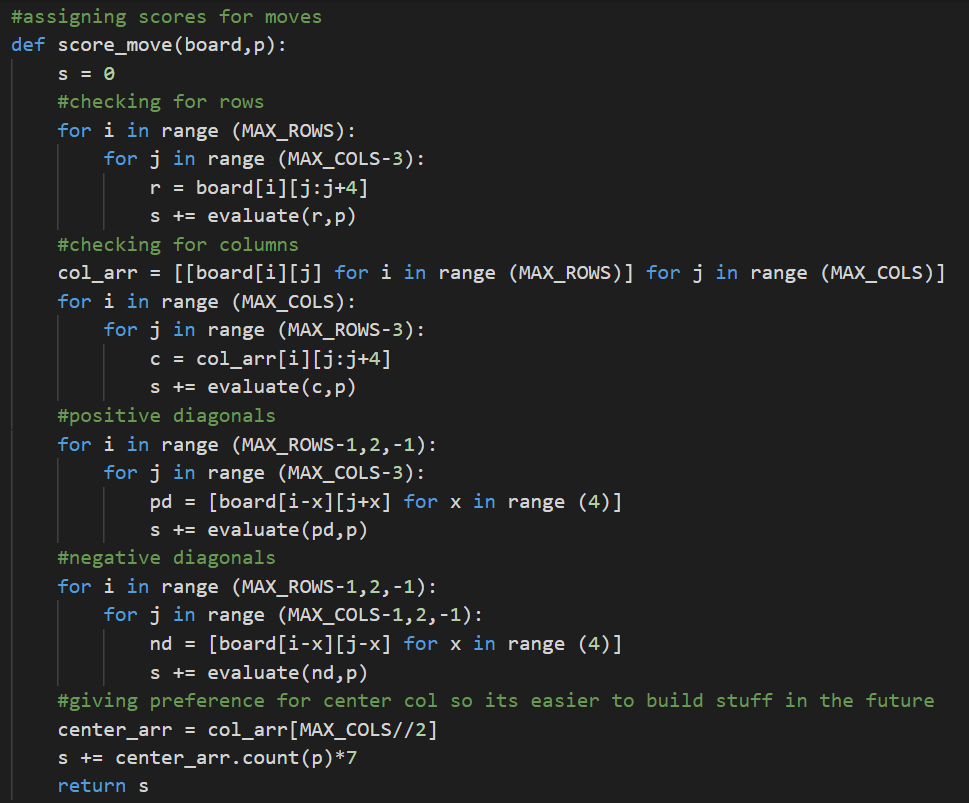The evaluate function assigns scores for a window passed to it depending on how many maximizing and minimizing tokens along with empty slots are present in the window, i.e, how favourable it is for the given player, and returns the score of that particular window.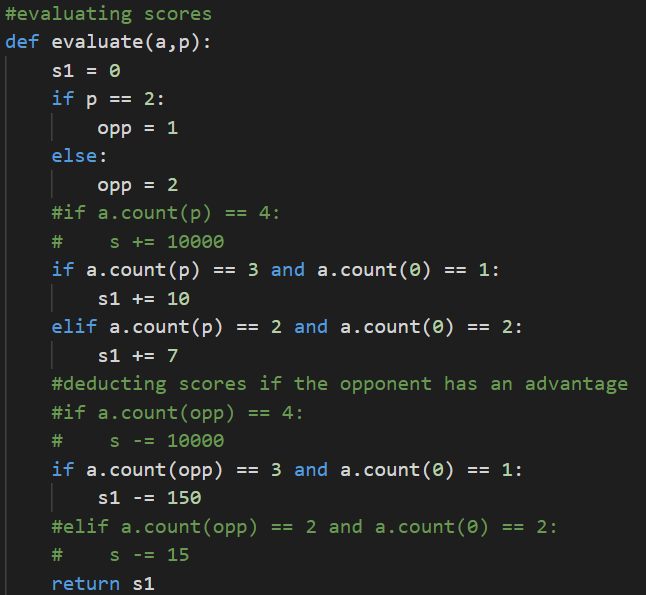## Alpha-Beta Pruning

The minimax algorithm searches all the nodes of the game tree up to a specific depth to find the best moves. The algorithm can be optimized to “prune” or exclude those branches of the game tree which are guaranteed to give worse moves.

### Core idea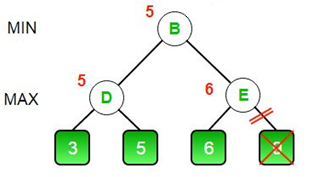Consider the above game tree. First, node D is evaluated and it is assigned the value of max(3,5) = 5. Now the algorithm begins evaluating node E. It evaluates the left branch and gets a value of 6. Since E is a maximizing node, its value will be greater than or equal to 6. However, node B is a minimizing node and its value will be the minimum of the values of nodes D and E. Since the value of node D is known to be 5, and E is at least 6, the value of the node B will be 5. Now the algorithm can prune or ignore the right branch of E, since it cannot influence the value of the node B. This is the core idea of the alpha beta pruning strategy.

### Implementation

To implement alpha beta pruning, we track the minimum score possible of the maximizing player in the alpha variable, and the maximum score possible of the minimizing player in the beta variable. If beta becomes less than or equal to alpha, we stop evaluating the descendants of the node.

Let us consider the previous example again. After evaluating node D, the minimizing player, at node B, is assured that the maximum possible score is 5. Any larger value is not possible since the minimizing player takes the minimum of possible scores. Hence, beta is 5.

At node E, upon evaluation of the left branch, the maximizing player is assured of a score of 6. Any smaller value is not possible since the maximizing player takes the maximum of possible scores. Hence, alpha becomes 6.

Since beta becomes less than alpha, we stop evaluating descendants of the node E.

In this manner, alpha beta pruning reduces the number of nodes evaluated, significantly improving the speed of minimax algorithm.

It has been implemented in the project in following manner: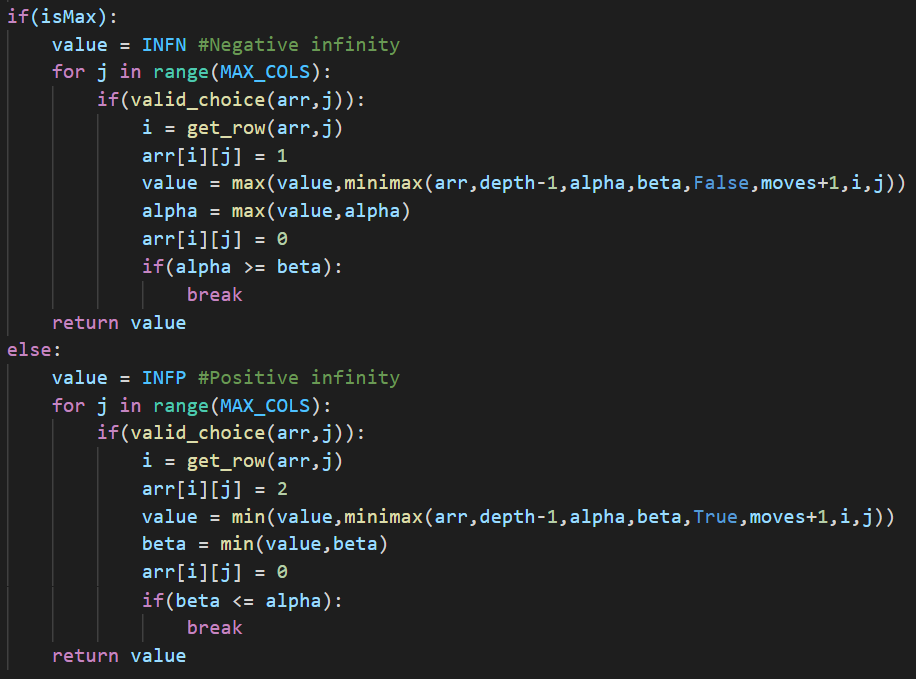## Pygame

Pygame is a free and open-source python library commonly used for making games. We have used pygame in our project to make the user interface.

### Handling inputs

The pygame.event module is used in the project to handle inputs from the user. The module stores all events such as mouse motion, mouse click, etc. in a queue. When pygame.event.get() is called, a list of events is returned and the event queue is reset. This list can be iterated and each event can be individually handled.

Each event has attributes associated with it such as event.pos, event.type, etc. which are used to discern the position of event, the type of event, etc.

An example of its use in the project is as follows: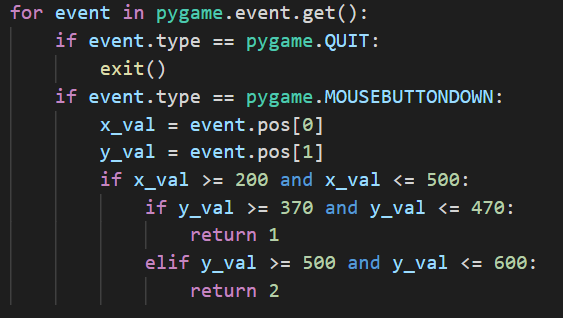In the above code, the list of events is iterated. For each event, we check the type of the event. Pygame.QUIT represents the action of pressing the close button and pygame.MOUSEBUTTONDOWN represents the action of clicking a mouse button. If the user clicks on the close button, the game terminates. If the user clicks somewhere else, we determine the position of the mouse click. The pos attribute of an event is a tuple containing the x and y coordinates of the event. If the mouse click lies in a particular region, the function returns a value.

### GUI

To draw the GUI and change it when required, we need a way to handle images. Pygame offers a way to do so in the pygame.Surface type object. Pygame.Surface contains useful attribute functions such as blit(), which is used to draw one surface over another.

We also need functions to manipulate these surfaces. For that purpose, draw and display modules in pygame are used.

The pygame.draw module contains functions to draw various shapes on a surface, and the pygame.display module lets us display a surface or multiple surfaces to the user.

Finally, we need a way to represent text in the game. The pygame.font module is used for rendering text on the screen. It uses pygame.font.Font type objects to store information about the style of text. This object also contains the render() attribute function to create a new surface with text written on it.

Let us take a look at an example where these are used in the project: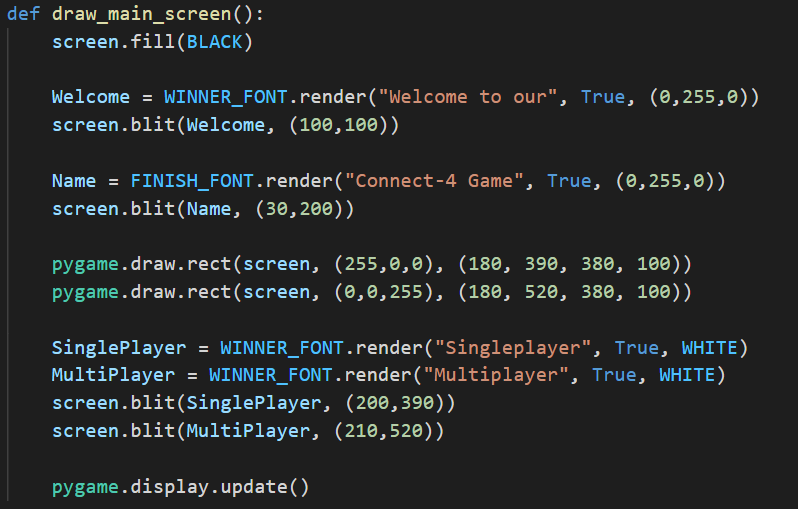Here, screen is the surface that is displayed to the user. WINNER_FONT and FINISH_FONT are font type objects defined as follows: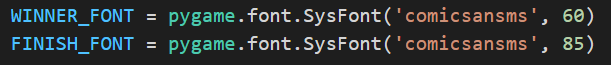Pygame.font.SysFont() is used to create a font type object. The two arguments represent the font style and size respectively.

First, screen.fill(BLACK) is called. This fills the display surface with the black colour.

Welcome and Name are surface type objects created using the render() function.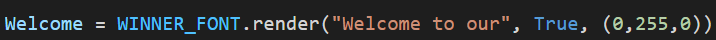The above line creates a surface that says “Welcome to our” in the text style represented by WINNER_FONT object. The colour of this text is (0,255,0) in RGB, i.e., green. The second argument is antialiasing, which is set to True to make the text appear smooth.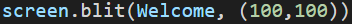The blit() function draws the Welcome surface over the screen surface at the specified position. This position is the position on the screen surface where the top left corner of the welcome surface will be placed.

The coordinates used are in pixels with origin at the top left corner of the screen surface. A coordinate of (x, y) is located x pixels to the right and y pixels down from the top left corner.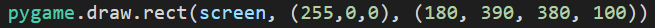Here, pygame.draw.rect() is used to draw a rectangle on the screen surface. (255,0,0) represents the colour of the rectangle in RGB, i.e., red. The next argument is a tuple which contains information about the position and size of the rectangle. The first two numbers, 180 and 390, are the x and y coordinates of the top left corner of the rectangle. The next two numbers, 380 and 100, are the width and height of the rectangle in pixels respectively.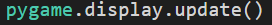All the changes made so far to the screen surface only affect the object, and are not displayed to the user. To update the screen seen by the user, we have to call the pygame.display.update() function.

The code given above finally generates the following screen:All the other screens of the game have been similarly created. Some of them are shown below: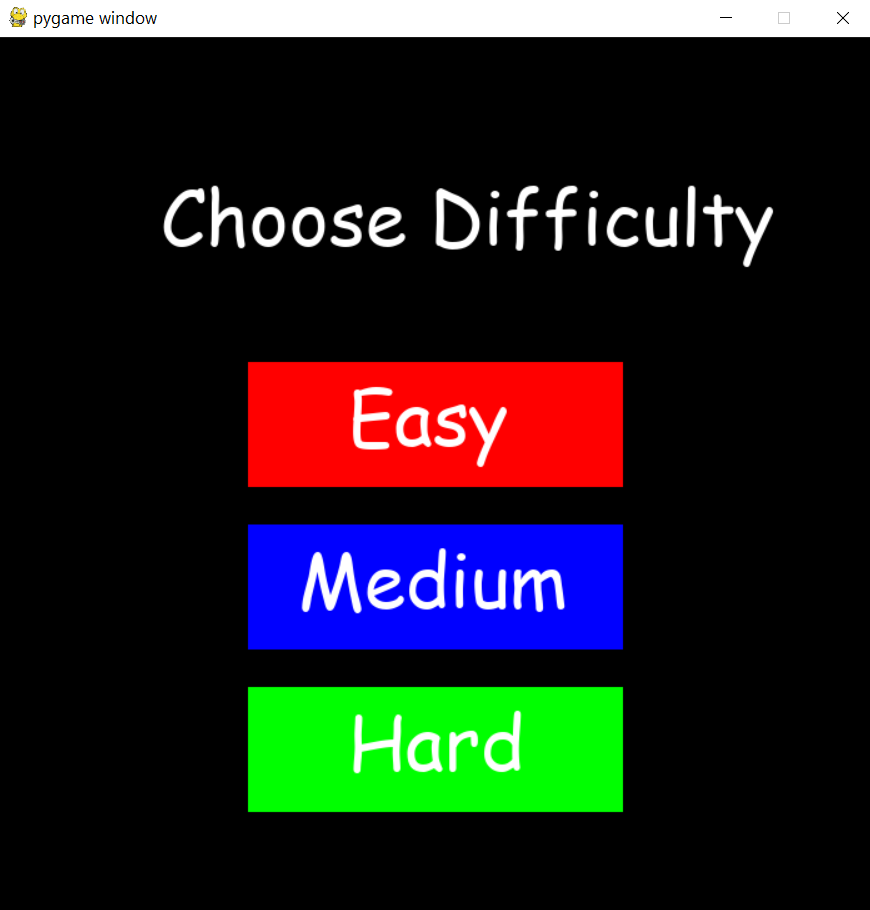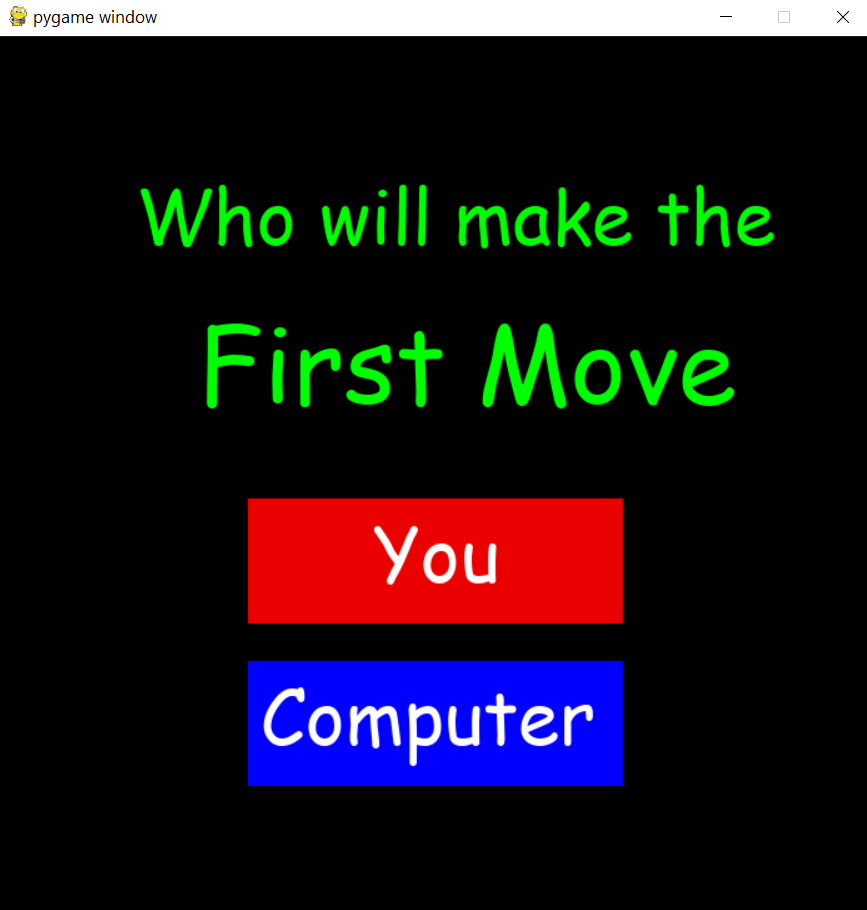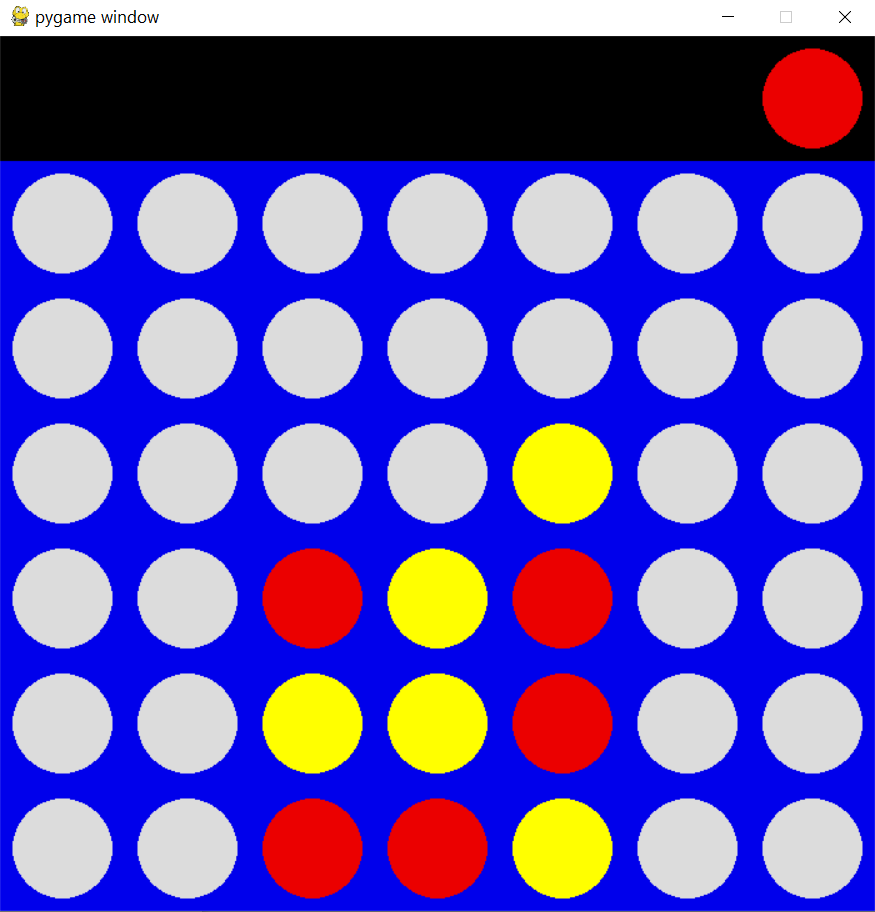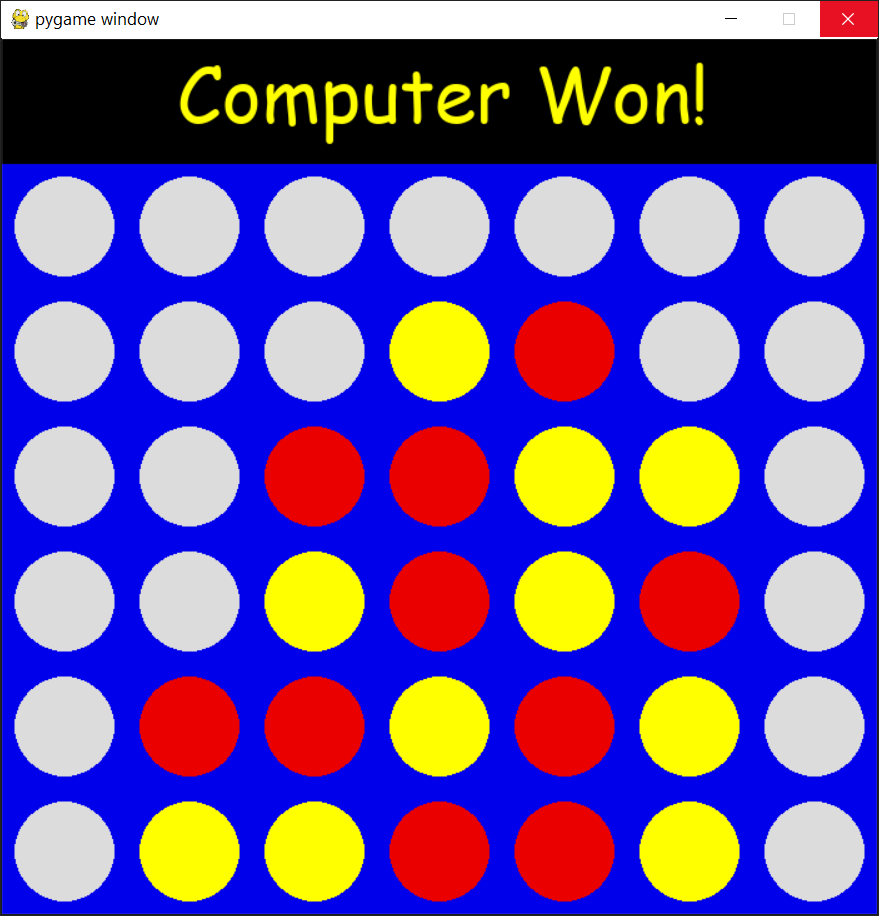## Conclusion

The end product is a fully functioning AI that can play a fairly decent game against any average human. However, there are some notable improvements that we can make in some of the functionality. Since a particular state of the board can be reached through more than one sequence of moves, it is evaluated multiple times as the algorithm does not store previous values. Another improvement that can be done is making the GUI more customizable and user friendly. Finally, additional features such as game review and game statistics can also be implemented in future iterations.